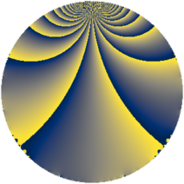# Properties

 Label 770.2.tLevel $770$ Weight $2$ Character orbit 770.t Rep. character $\chi_{770}(131,\cdot)$ Character field $\Q(\zeta_{6})$ Dimension $64$ Newform subspaces $2$ Sturm bound $288$ Trace bound $6$

# Related objects

## Defining parameters

 Level: $$N$$ $$=$$ $$770 = 2 \cdot 5 \cdot 7 \cdot 11$$ Weight: $$k$$ $$=$$ $$2$$ Character orbit: $$[\chi]$$ $$=$$ 770.t (of order $$6$$ and degree $$2$$) Character conductor: $$\operatorname{cond}(\chi)$$ $$=$$ $$77$$ Character field: $$\Q(\zeta_{6})$$ Newform subspaces: $$2$$ Sturm bound: $$288$$ Trace bound: $$6$$ Distinguishing $$T_p$$: $$13$$

## Dimensions

The following table gives the dimensions of various subspaces of $$M_{2}(770, [\chi])$$.

Total New Old
Modular forms 304 64 240
Cusp forms 272 64 208
Eisenstein series 32 0 32

## Trace form

 $$64q + 32q^{4} + 24q^{9} + O(q^{10})$$ $$64q + 32q^{4} + 24q^{9} - 6q^{11} + 12q^{14} + 16q^{15} - 32q^{16} - 8q^{22} - 24q^{23} + 32q^{25} + 60q^{26} - 24q^{33} + 48q^{36} - 8q^{37} - 24q^{38} + 6q^{44} - 24q^{47} - 40q^{49} + 16q^{53} - 8q^{58} - 120q^{59} + 8q^{60} - 64q^{64} - 48q^{66} - 8q^{67} + 32q^{71} - 40q^{77} + 32q^{78} - 48q^{82} - 16q^{86} - 4q^{88} - 72q^{89} + 88q^{91} - 48q^{92} + 40q^{93} - 44q^{99} + O(q^{100})$$

## Decomposition of $$S_{2}^{\mathrm{new}}(770, [\chi])$$ into newform subspaces

Label Dim. $$A$$ Field CM Traces $q$-expansion
$$a_2$$ $$a_3$$ $$a_5$$ $$a_7$$
770.2.t.a $$32$$ $$6.148$$ None $$0$$ $$0$$ $$0$$ $$0$$
770.2.t.b $$32$$ $$6.148$$ None $$0$$ $$0$$ $$0$$ $$0$$

## Decomposition of $$S_{2}^{\mathrm{old}}(770, [\chi])$$ into lower level spaces

$$S_{2}^{\mathrm{old}}(770, [\chi]) \cong$$ $$S_{2}^{\mathrm{new}}(77, [\chi])$$$$^{\oplus 4}$$$$\oplus$$$$S_{2}^{\mathrm{new}}(154, [\chi])$$$$^{\oplus 2}$$$$\oplus$$$$S_{2}^{\mathrm{new}}(385, [\chi])$$$$^{\oplus 2}$$